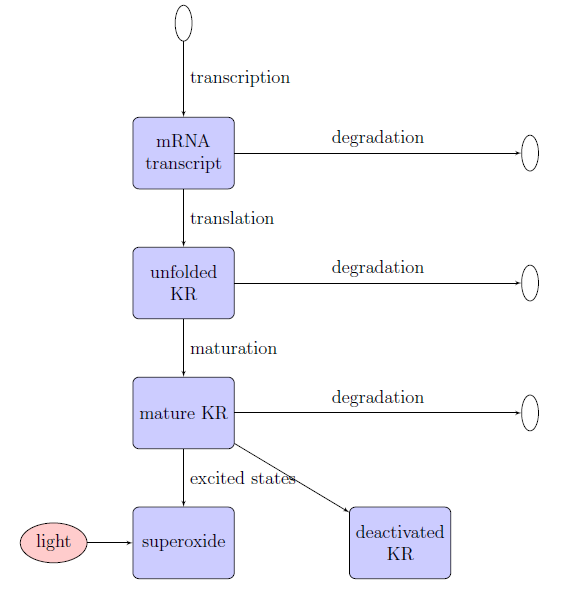Killer Red

# Intracellular model overviewThe intracellular model attempts to simulate the synthesis and action of KillerRed using ordinary differential equations over three stages of a typical photobleaching expeiment: induction at 37ºC, storage at 4ºC to allow for protein maturation, and photobleaching at room temperature. It includes:

• KillerRed synthesis, maturation, and degradation
• KillerRed states: singlet, singlet excited, triplet excited, and deactivated
• Superoxide production (by KillerRed)
• Superoxide elimination (by superoxide dismutase)

Parameters and assumptions:

• mRNA synthesis: adapted from Kennell's and Riezman's studies of the lac operon1. Our construct uses a wild-type lac promoter, so we assume that its transcription rates are similar to the lac operon.
• mRNA degradation: Characteristic half-life from Bernstein et. al.2
• KillerRed synthesis: Normal translation rates from Kennell and Riezman2. The maximum translation rate in the model is three orders of magnitude lower to reflect the presents of several rare codons. This adjustment is suggested by comparisons of our fluorescence data for KillerRed and mRFP, which have nearly identical brightness.
• Superoxide production: Given by calculations adapted from Tsien and Waggoner3; Song et. al.4; and our own fluorescence data. The photochemistry of the KillerRed chromophore is modeled after fluorescein photochemistry.

# Photochemistry of KillerRed fluorescence

Tsien et. al.3 give a method for determining $k_a$, the rate coefficient of activation from the ground state: $k_a = \sigma I$ where $\sigma$ is the optical cross-section per molecule and $I$ is the excitation intensity in photons per unit area. The lamp used for photobleaching gave $I=1\times 10^{27}$ photons/cm2s (about 1 W). $\sigma$ is given by $\sigma = \varepsilon (1000\text{cm}^3/\text{L})(\text{ln }10)N_A$ where $\varepsilon$ is the extinction coefficient and $N_A$ is Avogadro's number. We calculated $k_a = 1.72\times 10^{11}$ s-1 for KillerRed for our photobleaching experiments.

The rate constant for return to the ground state is obtained from $\tau$, the half life for KillerRed in the excited state (about 3.0 ns; value for dsRed, which has the same chromophore): $k_f = \frac{\text{ln }2}{\tau}$ $\tau$ for KillerRed is assumed to be similar to $\tau$ for dsRed (about 3.0 ns 5), since their chromophores are identical. Thus, $k_f = 2.3\times 10^{8}\text{ s}^{-1}$.

Using $k_a$ and $k_f$, the fraction $F_e$ of molecules in the excited state can be determined: $F = \frac{k_a}{k_a + k_f} = 0.9987$ Thus, we assume that KillerRed is always in the excited state (if it has not been deactivated) during photobleaching.

# Superoxide production

Production of the superoxide radical is governed by the following reactions. Fluorescein is used as a model chromophore4. $S$, $S^*$, $T^*$, and $Da$ are the singlet, excited singlet, excited triplet, and deactivated states, respectively, of the KillerRed chromophore. \begin{align*} S + h\nu \rightarrow S^* \qquad \qquad & k_a & \qquad \text{activation}\\ S^* \rightarrow S + h\nu \qquad \qquad & k_f & \qquad \text{deactivation by light emission}\\ S^* \rightarrow S \qquad \qquad & k_{ic}=6.9\times 10^{10} s^{-1} \qquad & \text{internal conversion} \\ S^* \rightarrow T^* \qquad \qquad & k_{isc}=6.6\times 10^6 s^{-1} \qquad & \text{intersystem crossing} \\ T^* \rightarrow S^* \qquad \qquad & k_{rd}=50 s{-1} \qquad & \text{radiationless deactivation} \\ T^* + 2H_2O \rightarrow S + O_2^{\bullet -} \qquad \qquad & k_{s1}=c\frac{k_{photobleach}}{\Phi} \qquad &\text{superoxide production w/o deactivation} \\ T^* + 2H_2O \rightarrow Da + O_2^{\bullet -} \qquad \qquad & k_{s2}=\frac{k_{photobleach}}{\Phi} \qquad &\text{superoxide production with deactivation} \\ \end{align*} Here, $k_a$ is the activation constant (defined above), and $k_f$ is the fluorescence constant (defined above). $k_{ic}$, $k_{isc}$, and $k_{rd}$ were obtained from Song et. al.4. $k_{photobleach}$ is the photobleaching (or photofading) constant obtained from our experimental fluorescence data, $\Phi$ is the quantum yield, and $c$ is a constant that reflects the relative propensity for KillerRed to generate superoxide without becoming deactivated.

## Superoxide dismutase

Superoxide dismutase is E. coli's main defense against superoxide. Its action was incorporated using Michaelis-Menten kinetics: $-\frac{d[O_2^{\bullet -}]}{dt} = \frac{V_{\text{max}}[O_2^{\bullet -}]}{K_m + [O_2^{\bullet -}]}$ $V_{max}$ was estimated using $k_{cat}$ from Karadag and Bilgin6, and $k_m$ was estimated using $k_m$ from the same and $k_{cat}/k_m$ from Falconi et. al.7

# Matlab functions

• incubate37.m: The first part of the experiment, in which the culture is induced with IPTG and incubated at 37$^{\circ}$C. Transcription rate is high, and KillerRed excitation is low (since cultures were covered to block light).
• incubate04.m: The second part of the experiment, in which the culture is incubated at 4$^{\circ}$C so that the KillerRed chromophore can mature. Most reaction rates are considerably lower.
• photobleach.m: The final part of the experiment, in which the culture is exposed to a high amount of light so that the KillerRed chromophore can mature. Here, amount of KillerRed with a chromophore in the ground state is considered to be negligible.